Name:    Chapter 11 Post Test

Multiple Choice
Identify the choice that best completes the statement or answers the question.

1.

Evaluate the function as indicated. Round to three decimal places if necessary.a. 10,303.010 b. 10,936.853 c. 91,999.387 d. 4702.847 e. 90,900.000

2.

Evaluate the function as indicated. Round your answer to three decimal places.a. –9.473 b. 10.705 c. –169.829 d. 9.473 e. 517.234

3.

Identify the horizontal asymptote of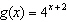.
 a. x-axis b.c.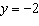d.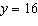e.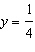4.

Determine the principal P (to the nearest cent) that will yield a balance ofdollars when invested at rate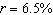for 40 years, compounded 12 times per year. Round the answer to two decimal places.
 a. \$973.96 b. \$273,927.55 c. \$16,025.64 d. \$37,398.27 e. \$402,834.63

5.

Determine the principal P (to the nearest cent) that will yield a balance of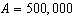dollars when invested at ratefor 40 years, compounded continuously. Round the answer to two decimal places.
 a. \$611,887.72 b. \$495,364.63 c. \$16,686.63 d. \$13,015.58 e. \$13,608.96

6.

Find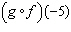where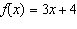and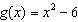.
 a. 73 b. 115 c. 223 d. 61 e. –9

7.

Find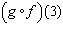where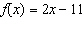and.
 a. –16 b. 2 c. 1 d. –15 e. –5

8.

Find the domain of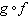whereand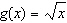.
 a.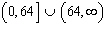b.c.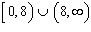d.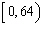e.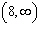9.

Which statement(s) verify that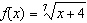and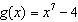are inverse?
 a.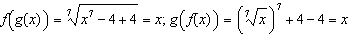b.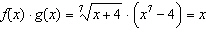c.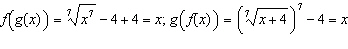d.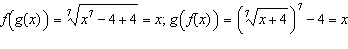e.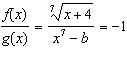10.

Write the logarithmic equation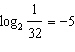in exponential form.
 a.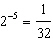b.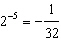c.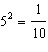d.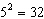e.11.

Use the properties of logarithms to evaluate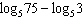without a calculator.
 a. 2 b.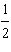c.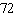d.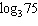e. None of these

12.

Use the properties of logarithms to evaluatewithout a calculator.
 a. 0 b. 5 c.d.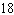e. 12

13.

Use the properties of logarithms to evaluate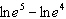without a calculator.
 a.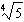b.c. 0 d.e.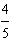14.

Use the properties of logarithms to expand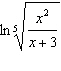.
 a.b.c.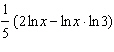d.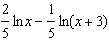e.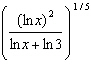15.

Use the properties of logarithms to condense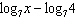.
 a.b.c.d.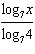e.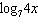16.a.b.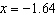c.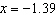d.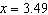e.17.a.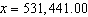b.c.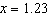d.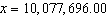e.18.

An investment of \$2500 is made in an account that compounds interest quarterly. After years 30  the balance in the account is \$26132.78. To the nearest tenth of a percent, what is the annual interest rate for this account?
 a.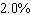b.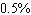c.d.e.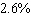19.

An investment of \$28,000 is made in a trust fund at an annual interest rate of 6.75%, compounded continuously. How long will it take for the investment to double? Give your answer to two decimal places.
 a.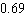year b.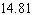years c.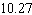years d.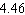years e.years

20.

An investment is made in an account that pays 10%, compounded monthly. What is the effective yield for this investment? Round your answer to two decimal places.
 a. 10.52% b. 10.00% c. 10.38% d. 10.25% e. 10.47%